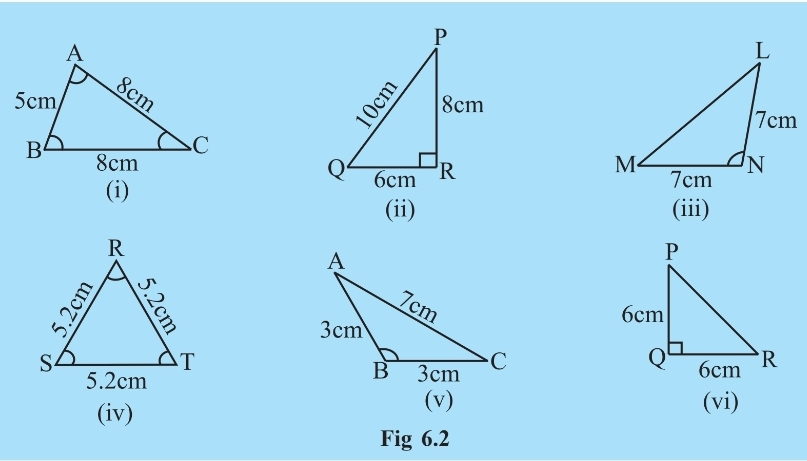# 3.  Look at Figand classify each of the triangles according to its            (a) Sides            (b) Anglesi) The triangle ABC

Based on Side: In Triangle ABC, Since two sides (BC and AC ) are equal (= 8 cm ) The given triangle is an isosceles triangle.

Based on Angle: In Triangle ABC, Since all the triangles are less than 90 degrees, So the given triangle is Acute angled triangle.

ii) The Triangle PQR

Based on Side: In Triangle PQR, All the sides are different so, The given triangle is a scalene triangle.

Based on Angle: In Triangle PQR, Since angle QRP is a right angle, So the given triangle is Right-angled triangle.

iii)The Triangle LMN

Based on Side: In Triangle LMN, Since two sides (MN and NL ) are equal (=7 cm ), The given triangle is an isosceles triangle.

Based on Angle: In Triangle LMN, Since angle MNL is an Obtuse angle, So the given triangle is Obtuse angled triangle.

iv) The Triangle RST

Based on Side: In Triangle RST, All the sides are equal (=5.2 cm) so, The given triangle is an Equilateral triangle.

Based on Angle: In Triangle RST, Since all the triangles are less than 90 degrees, So the given triangle is Acute angled triangle.

v)The triangle ABC

Based on Side: In Triangle ABC, Since two sides (AB and BC ) are equal (= 3 cm ) The given triangle is an isosceles triangle.

Based on Angle: In Triangle ABC, Since angle ABC is greater than 90 degrees  So the given triangle is Obtuse angled triangle.

vi)The Triangle PQR

Based on Side: In Triangle PQR, Since two sides (PQ and QR ) are equal (= 6 cm ) The given triangle is an isosceles triangle.

Based on Angle: In Triangle PQR, Since angle PQR is a right angle, So the given triangle is Right-angled triangle.

## Related Chapters

### Preparation Products

##### Knockout NEET 2024

Personalized AI Tutor and Adaptive Time Table, Self Study Material, Unlimited Mock Tests and Personalized Analysis Reports, 24x7 Doubt Chat Support,.

₹ 40000/-
##### Knockout NEET 2025

Personalized AI Tutor and Adaptive Time Table, Self Study Material, Unlimited Mock Tests and Personalized Analysis Reports, 24x7 Doubt Chat Support,.

₹ 45000/-
##### NEET Foundation + Knockout NEET 2024

Personalized AI Tutor and Adaptive Time Table, Self Study Material, Unlimited Mock Tests and Personalized Analysis Reports, 24x7 Doubt Chat Support,.

₹ 54999/- ₹ 42499/-
##### NEET Foundation + Knockout NEET 2024 (Easy Installment)

Personalized AI Tutor and Adaptive Time Table, Self Study Material, Unlimited Mock Tests and Personalized Analysis Reports, 24x7 Doubt Chat Support,.

₹ 3999/-